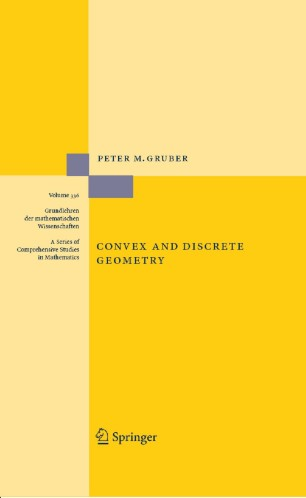# Convex and Discrete Geometry

• Peter M. GruberBook

Part of the A Series of Comprehensive Studies in Mathematics book series (GL, volume 336)

1. Front Matter
Pages I-XIII
2. Pages 1-38
3. Pages 39-242
4. Pages 243-351
5. Pages 353-512
6. Back Matter
Pages 513-580

### Introduction

Convex and Discrete Geometry is an area of mathematics situated between analysis, geometry and discrete mathematics with numerous relations to other areas. The book gives an overview of major results, methods and ideas of convex and discrete geometry and its applications. Besides being a  graduate-level introduction to the field, it is a practical source of information and orientation for convex geometers. It should also be of use to people working in other areas of mathematics and in the applied fields.

### Keywords

Convex body combinatorial geometry convex function convex polytope discrete geometry geometric lattice

#### Authors and affiliations

• Peter M. Gruber
• 1
1. 1.Institute of Discrete Mathematics and GeometryVienna University of TechnologyViennaAustria